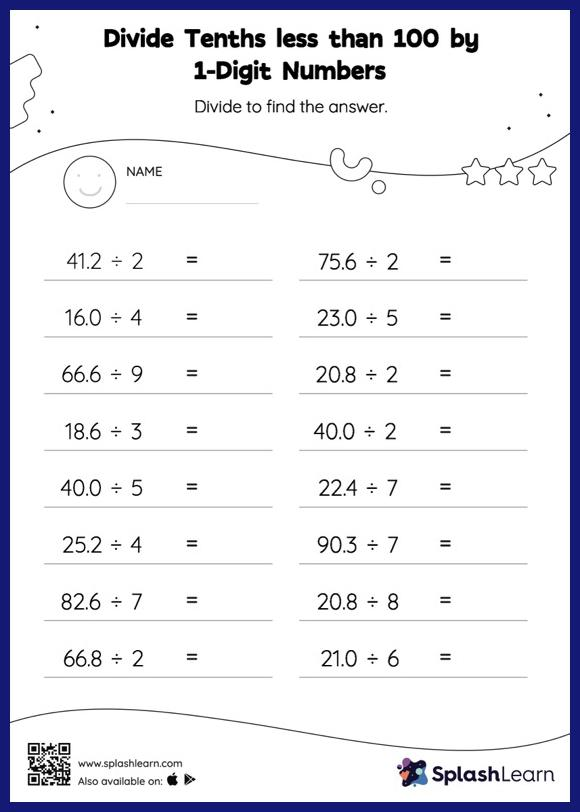# Divide Tenths less than 100 by 1-Digit Numbers without Remainder: Horizontal Division Worksheet

Home > Divide Tenths less than 100 by 1-Digit Numbers without Remainder: Horizontal DivisionThis worksheet invites students to divide tenths less than 100 by 1-digit numbers without remainder, which helps them become proficient in division. When dividing decimals by 1-digit numbers, students first divide the same way they would divide with whole numbers. Then they add a decimal point at the correct place in the quotient depending on the number of digits in the dividend. Divide tenths less than 100 by 1-digit numbers without remainder worksheet helps them improve accuracy with this concept.